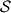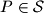### IMO Shortlist 2011 problem C3

Kvaliteta:
Avg: 0.0
Težina:
Avg: 7.0
Let$\mathcal{S}$ be a finite set of at least two points in the plane. Assume that no three points of$\mathcal S$ are collinear. A windmill is a process that starts with a line$\ell$ going through a single point$P \in \mathcal S$. The line rotates clockwise about the pivot$P$ until the first time that the line meets some other point belonging to$\mathcal S$. This point,$Q$, takes over as the new pivot, and the line now rotates clockwise about$Q$, until it next meets a point of$\mathcal S$. This process continues indefinitely.
Show that we can choose a point$P$ in$\mathcal S$ and a line$\ell$ going through$P$ such that the resulting windmill uses each point of$\mathcal S$ as a pivot infinitely many times.

Proposed by Geoffrey Smith, United Kingdom
Source: Međunarodna matematička olimpijada, shortlist 2011